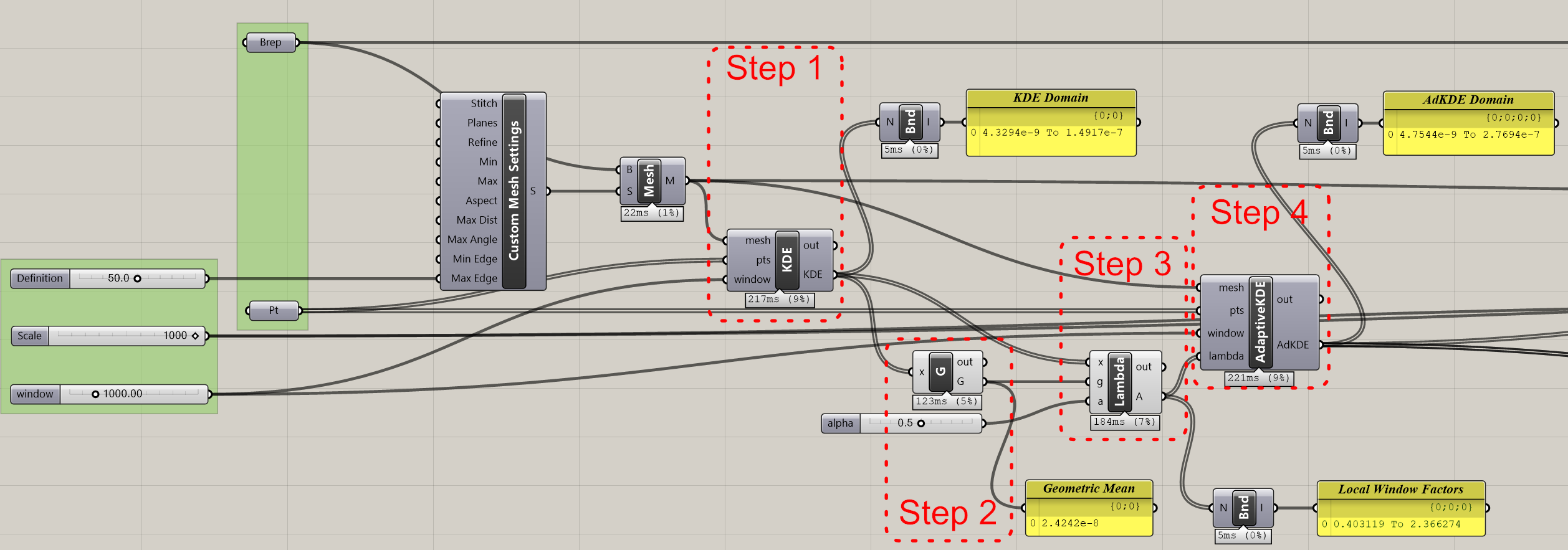Category : Code, Research

Adaptive KDE is an improvement of a simple KDE. Selecting a window that adequately smooths all the data can be a difficult if not impossible. Frequently, it is necessary to compromise on the smoothness of the resulting estimate on the areas of low density. Adaptive KDE attempts to address the problem by allowing the window or bandwidth to vary. In areas of larger concentration of points the window becomes smaller, and in areas of lower concentration the window becomes larger. As the density is unknown, the first step is to do a pilot estimation using a normal KDE function. Using this density we then calculate the geometric mean of the density values for each point. This value is used to calculate a lamba factor which is then used in the adaptive kernel function to calculate locally adjusted estimates. The steps can be summarized as follows (Silverman 1986):

1. Find a pilot estimate kde(x) that satisfies kde(Xi)>0 for all i;
2. Calculate the geometric mean of kde(Xi);
3. Calculate the local window factor lambda for each i;

The Grasshopper definition looks as follows:Step 1: For the first step any kde function can be used. But it must be assured that all vertices of the analysis mesh have estimates larger than 0, ie the domain of all the numbers can’t start in zero or bellow.

Step 2: Grasshopper already has a geometric mean component. But it seems to use a mass multiplication, which likely won’t work with estimations, since these can be very small numbers. The C# code bellow uses a different method of calculating the geometric mean.

```private void RunScript(List x, ref object A)
{
double tempv = 0.0;
double a = 0.0;
for (int i = 0; i < x.Count; i++)
tempv += Math.Log(x[i]);

a = tempv / x.Count;

if(Double.IsNaN(a))
return;

if(Double.IsInfinity(a))
return;

A = Math.Pow(Math.E, a);

}```

Step 3: The code below requires the input of an a parameter, with a value between 0 and 1. And adequate value is 0,5. If 0 is used, the function will revert back to a simple KDE.

```private void RunScript(List x, double g, double a, ref object A)
{
if (g <= 0)
return;
if (a < 0 || a > 1)
return;
if (x.Count == 0)
return;

List lambda = new List();
for(int i = 0; i < x.Count; i++)

A = lambda;
}```

Step 4: The last step is to actually calculate the estimate.

```private void RunScript(Mesh mesh, List pts, double window, List lambda, ref object A)
{
var rtnlist = new List();
for(int i = 0; i < mesh.Vertices.Count; i++)
{
double l = lambda[i];
double tempval = 0.0;
double kde = 0.0;
Point3d v = new Point3d(mesh.Vertices[i]);
for(int j = pts.Count - 1; j > 0; j--)
{
var pt = pts[j];
Vector3d vt = new Vector3d(v - pt);
tempval += kernel(vt / (window * l)) / ((window * window) * (l * l));
}
kde = 1.0 / pts.Count * tempval;
}

A = rtnlist;
}

public double kernel(Vector3d input)
{
//Epanechnikov Kernel
double k = Vector3d.Multiply(input, input);
if ( k < 1)
{
return 3 * (1 / Math.PI) * (1 - k) * (1 - k);
}else{
return 0;
}
}```